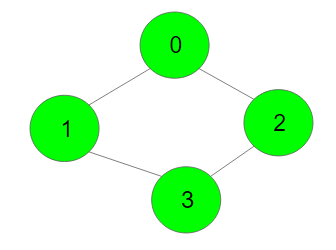XDAYS

:

HOUR

:

MINS

:

SEC

Copied to Clipboard
Euler Circuit in an Undirected Graph
Medium Accuracy: 54.36% Submissions: 4299 Points: 4

Eulerian Path is a path in a graph that visits every edge exactly once. Eulerian Circuit is an Eulerian Path that starts and ends on the same vertex. Given the number of vertices V and adjacency list adj denoting the graph. Your task is to find that there exists the Euler circuit or not

Note that: Given graph is connected.

Example 1:

Input:Output: 1
Explanation: One of the Eularian circuit
starting from vertex 0 is as follows:
0->1->3->2->0


You don't need to read or print anything. Your task is to complete the function isEularCircuitExist() which takes V and adjacency list adj as input parameter and returns boolean value 1 if Eular circuit exists otherwise returns 0.

Expected Time Complexity: O(V + E)
Expected Space Complexity: O(V)

Constraints:
1 <= V <= 105
1 <= E <= 2*105

We are replacing the old Disqus forum with the new Discussions section given below.

### Editorial

We strongly recommend solving this problem on your own before viewing its editorial. Do you still want to view the editorial?

#### My Submissions: